The this keyword can sometime be one of the most confusing things of JavaScript, as it is different then what in other object oriented languages like C++, Java.

It is such a big topic, that if you need to master it you need to go through a whole book by Kyle Simpson — You don’t know JS: this & object prototypes. You can find the free version of the book here.

We will understand this in JavaScript first then will move to the this.bind for react.

## Understanding “this” in JS

Objects are the basics of JavaScript, even functions are object in JavaScript. Now “this” is a special object in JavaScript and it’s value is decided on how the code is executed.

### “this” in simple function

In a normal JS function like below, the “this” refers to global window object.

``````function foo() {
console.log(this);
console.log(this === window);
}

foo();
``````

Running the above code will give.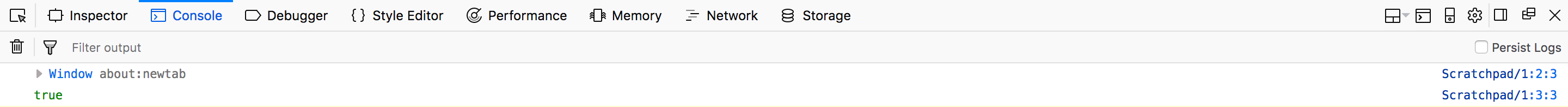global window object

There is a special case with strict mode.

In strict mode “this” to be undefined , as global object refers to undefined instead of window object.

``````function foo() {
'use strict';
console.log(this);
console.log(this === window);
}

foo();
``````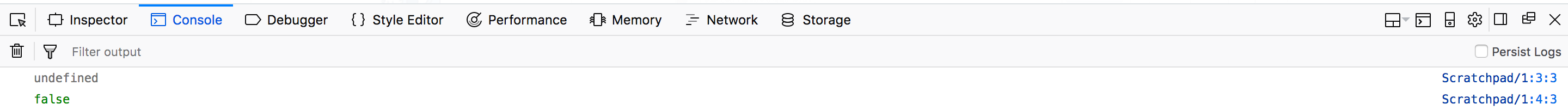this is undefined

### “this” in a object property

In a object which have a property as a function, the value of “this” is the Object itself.

``````var obj = {};
obj.foo = function() {
console.log("Inside obj foo");
console.log(this);
}

obj.foo();
``````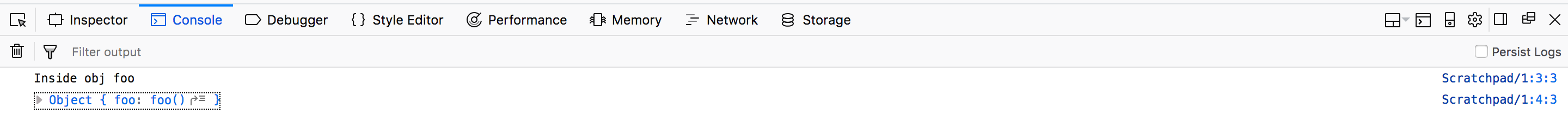this is Object itself

### “this” with constructor function

When a function is called with “new” keyword, it is know as constructor function. And the value of “this” refers to the newly created instance.

``````function Person(fn, ln) {
this.first_name = fn;
this.last_name = ln;

this.displayName = function() {
console.log(
``````

`Name: \${this.first_name} \${this.last_name}` ); console.log(this); } }

``````let person = new Person("Nabendu", "Biswas");
person.displayName();
let person2 = new Person("Shikha", "Biswas");
person2.displayName();
``````

The newly created instance is nothing but an object, because everything in JS is a object.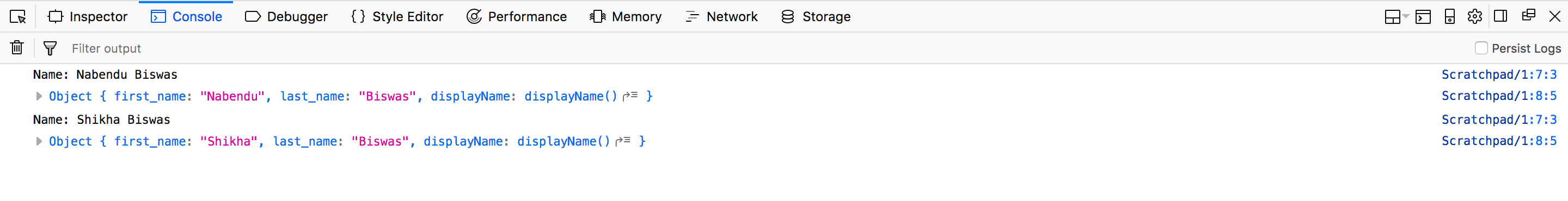this refers to newly created Object

### “this” with call, apply and bind method

Almost everything in JavaScript is an object,including functions. Every functions have call, apply and bind methods. These methods can be used to set the custom value of “this” to the execution context of the function.

### call

Let’s say that we have an object called obj. It only has one property called num, which has a value of 3. Let’s also make a function called addNumbers.

``````let obj = {num: 3};
let addNumbers = function(a, b, c){
console.log(this);
return this.num + a + b + c;
};
``````

Now, in addNumbers we have this.num. But how do we pass the value obj.num to it. We need to pass it a context, which means the value of “this”. We will do this my call method by passing a first argument as obj, so the “this” is the obj now.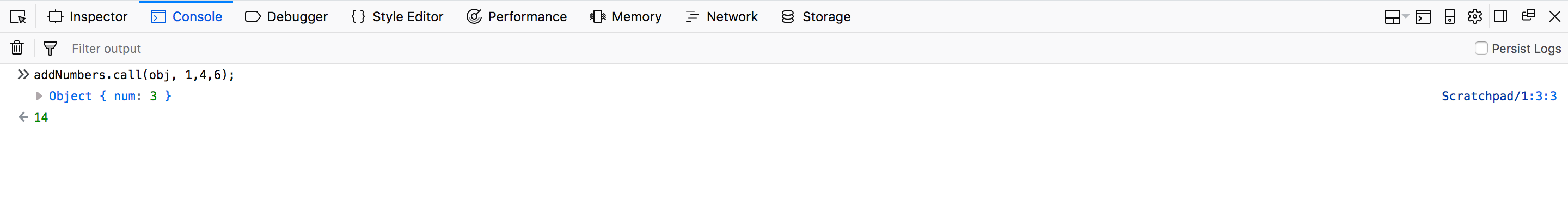this is obj

### apply

It is totally similar to call, but the only difference is that we can pass array as the second argument. We will use the same code for apply also.

``````let obj = {num: 3};
let addNumbers = function(a, b, c){
console.log(this);
return this.num + a + b + c;
};
``````

But now we have an arr, to be passed as second argument.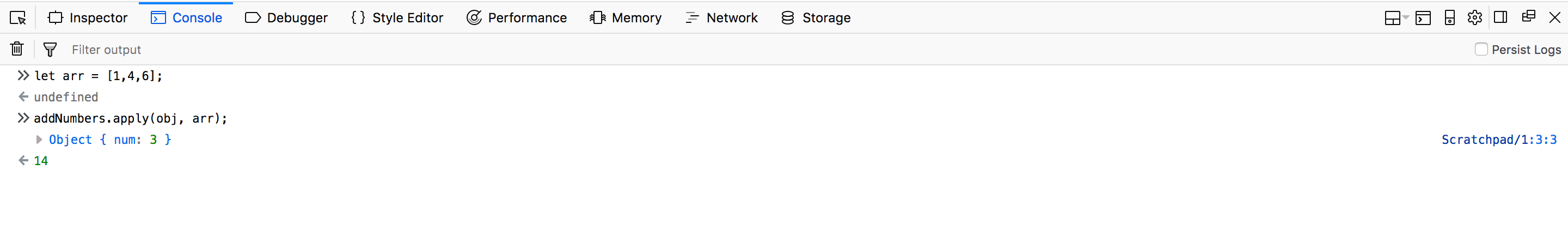arr as second argument

### bind

Bind works in a bit different way then call and apply. It works by returning a copy of the function. So, we will use the same code.

``````let obj = {num: 3};
let addNumbers = function(a, b, c){
console.log(this);
return this.num + a + b + c;
};
``````

We will take the returned function in bindFunc and then execute it in the next line.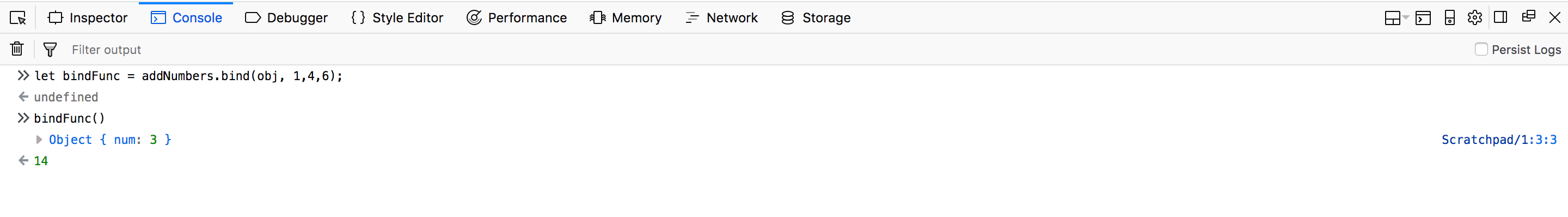bind explained

## Understanding “this” in React

Anyone who have worked with React, must have used the bind function inside the constructor for a function which was called through an input event.

``````class Foo extends React.Component{
constructor( props ){
super( props );
this.state = {
text: ''
}

this.handleChange = this.handleChange.bind(this);
}

handleChange(event){
this.setState({
text: event.target.value
})
}

render(){
return (
<input type="text" value={this.state.text}
**onChange={this.handleChange}** />
);
}
}

ReactDOM.render(
<Foo />,
document.getElementById("app")
);
``````

If we don’t give the line this.handleChange = this.handleChange.bind(this), then handleChange() will throw error that

``````'this' is undefined
``````

This issue occurs because of JavaScript and not React. Let’s recreate this issue in plain JavaScript. Running the code in Firefox scratchpad gives error.

``````class Foo {
constructor(name){
this.name = name
}
display(){
console.log(this.name);
}
}
var foo = new Foo('Nabendu');
foo.display(); //Outputs Nabendu

var display = foo.display;
display();
***/*
Exception: TypeError: this is undefined
*/***
``````

The assignment operation below simulates loss of context, similar to passing the handler as a callback in the actual React Component.

This gives undefined because the bodies of classes are by default in strict mode and in strict mode the global object is undefined.

To prevent this error we need to bind “this” value.

``````class Foo {
constructor(name){
this.name = name;
this.display = this.display.bind(this);
}
display(){
console.log(this.name);
}
}
var foo = new Foo('Nabendu');
foo.display(); //Outputs Nabendu

var display = foo.display;
display();  //Outputs Nabendu
``````

The same thing happens in React. When we bind the “this” of the event handler to the component instance in the constructor, we can pass it as a callback without worrying about it losing its context.

### “arrow” function in the callback

We can also avoid the bind statement by using arrow function in callback.

``````class Foo extends React.Component{
constructor( props ){
super( props );
this.state = {
text: ''
}
}

handleChange(event){
this.setState({
text: event.target.value
})
}

render(){
return (
<input type="text" value={this.state.text}
**onChange={(e) => this.handleChange(e)}** />
);
}
}

ReactDOM.render(
<Foo />,
document.getElementById("app")
);
``````

The reason is that in the case of arrow functions, “this” is bound lexically. This means that it uses the context of the enclosing function — or global — scope as its “this” value.